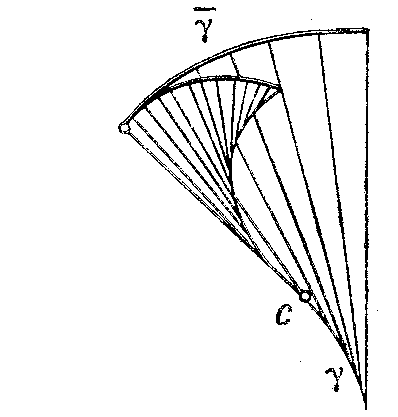# Evolvent of a plane curve

2010 Mathematics Subject Classification: Primary: 53A04 [MSN][ZBL]

involute

A curve $\bar\gamma$ assigned to the plane curve $\gamma$ such that $\gamma$ is the evolute of $\bar\gamma$. If $\mathbf{r} = \mathbf{r}(s)$ (where $s$ is the arc length parameter of $\gamma$) is the equation of $\gamma$, then the equation of its evolvent has the form $$\bar{\mathbf{r}} = \mathbf{r}(s) + (c-s)\tau(s) \,,$$ where $c$ is an arbitrary constant and $\tau$ the unit tangent vector to $\gamma$. The figures show the construction of the evolvent in two typical cases: a) if for any $s<c$ the curvature $k(s)$ of $\gamma$ does not vanish (the evolvent is a regular curve); and b) if $k(s)$ vanishes only for $s=s_1$ and $k'(s_1) \ne 0$ (the point corresponding to $s=s_1$ on the evolvent is a cusp of the second kind).Figure: e036720aFigure: e036720b

About the evolvent of a surface, see Evolute (surface).